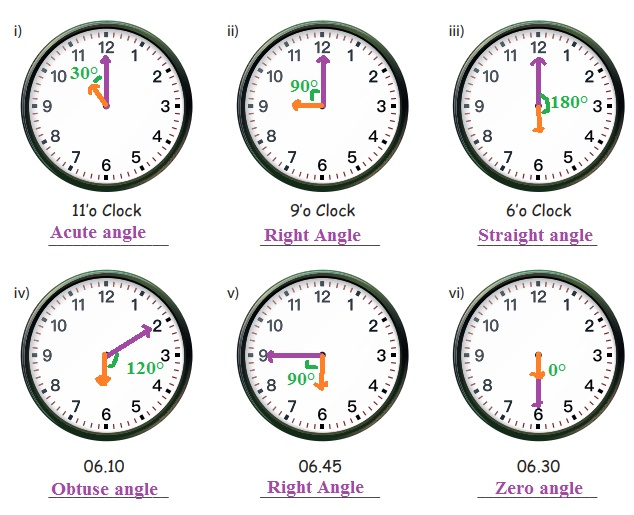Home | | Maths 5th Std | Exercise 3.2 (Rotating angles)

# Exercise 3.2 (Rotating angles)

Text Book Back Exercises Questions with Answers, Solution : 5th Maths : Term 2 Unit 3 : Patterns : Exercise 3.2 (Rotating angles)

Exercise 3.2

1. Mention the time in the clock when the angle is

i) 180°, ii) 90°, iii) 60°2. Find the angle made by the hands of the clock at the given time.Tags : Patterns | Term 2 Chapter 3 | 5th Maths , 5th Maths : Term 2 Unit 3 : Patterns
Study Material, Lecturing Notes, Assignment, Reference, Wiki description explanation, brief detail
5th Maths : Term 2 Unit 3 : Patterns : Exercise 3.2 (Rotating angles) | Patterns | Term 2 Chapter 3 | 5th Maths